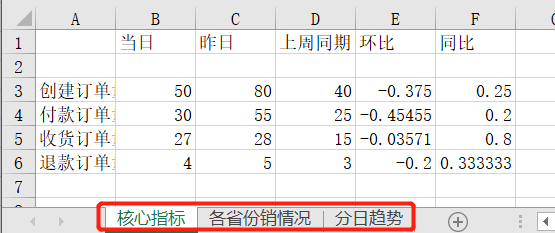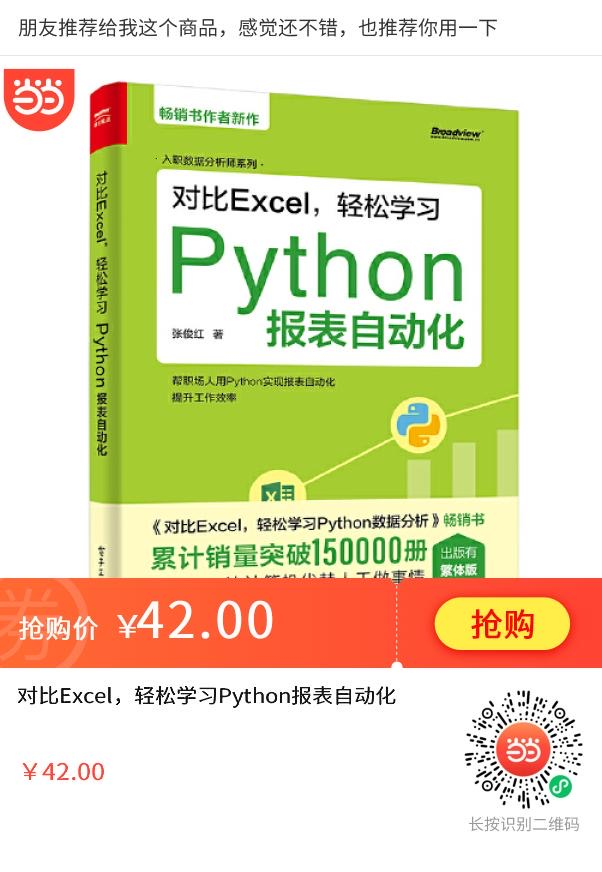#### 对比Excel，轻松学习Python报表自动化实战！

1.Excel的基本组成
2.一份报表自动化的流程
3.报表自动化实战
- 当日各项指标同环比情况
- 当日各省份创建订单量情况
- 最近一段时间创建订单量趋势
4.将不同的结果进行合并
- 将不同结果合并到同一个Sheet中
- 将不同结果合并到同一个工作簿的不同Sheet中

# Excel的基本组成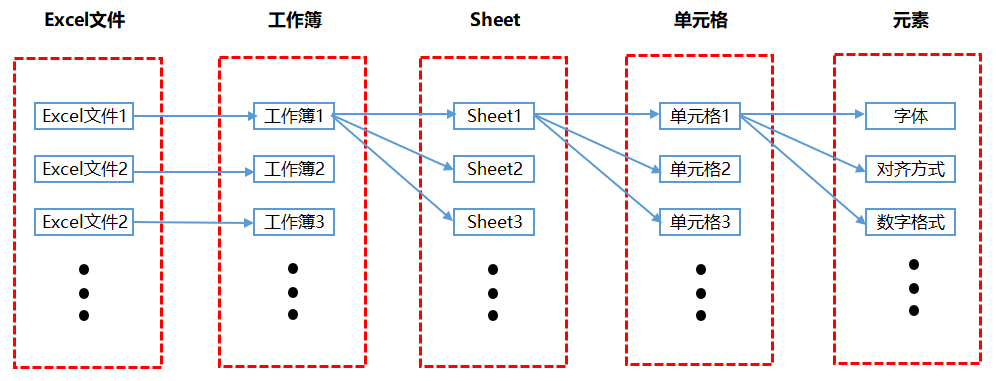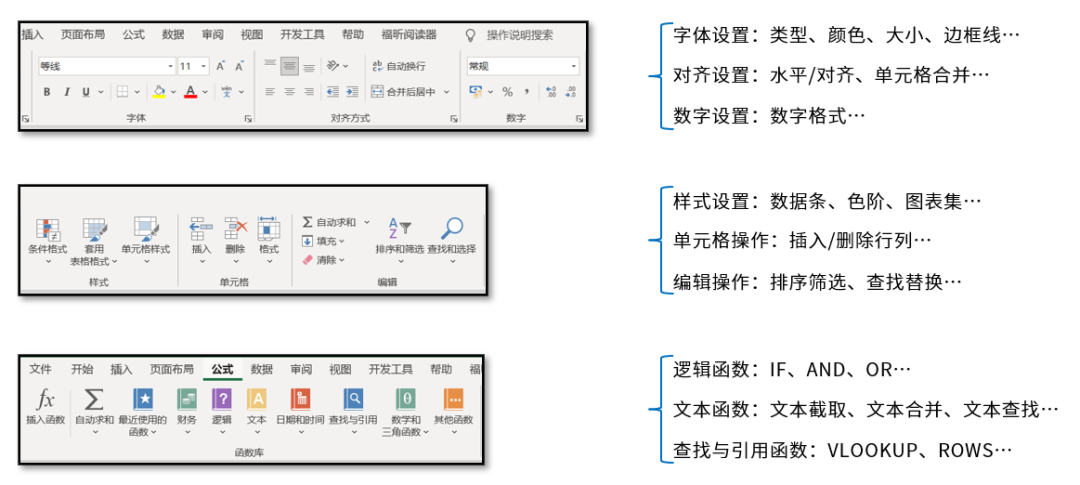# 一份自动化报表的流程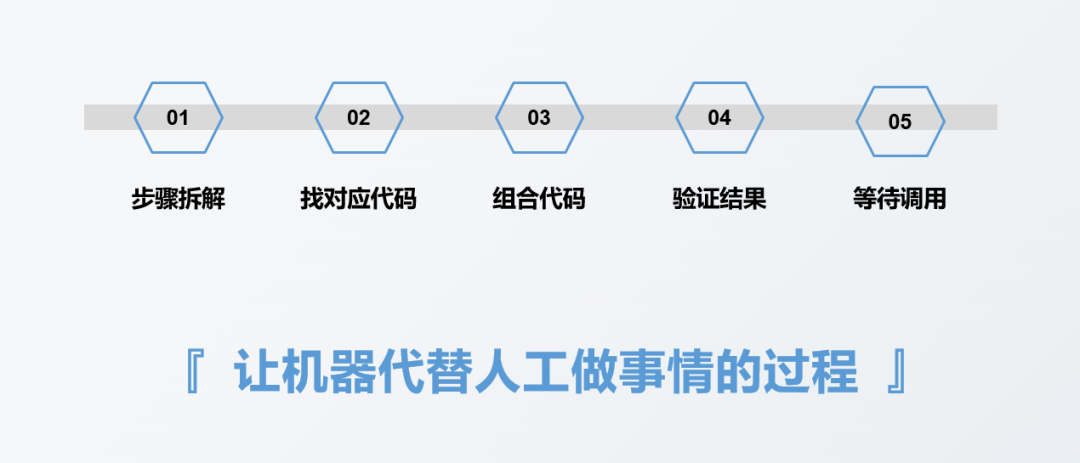# 报表自动化实战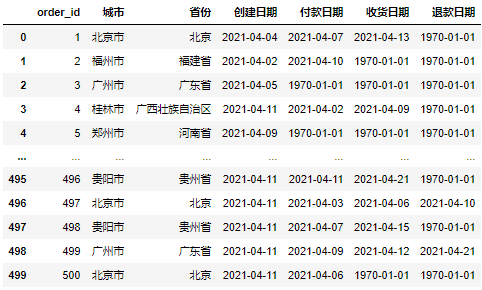• 当日各项指标的同环比情况；
• 当日各省份创建订单量情况；
• 最近一段时间创建订单量趋势

## 当日各项指标的同环比情况：

``#导入文件import pandas as pddf = pd.read_excel(r'D:\Data-Science\share\excel-python报表自动化\sale_data.xlsx')#构造同时获取不同指标的函数def get_data(date):       create_cnt = df[df['创建日期'] == date]['order_id'].count()    pay_cnt = df[df['付款日期'] == date]['order_id'].count()    receive_cnt = df[df['收货日期'] == date]['order_id'].count()    return_cnt = df[df['退款日期'] == date]['order_id'].count()    return create_cnt,pay_cnt,receive_cnt,return_cnt    #假设当日是2021-04-11#获取不同时间段的各指标值df_view = pd.DataFrame([get_data('2021-04-11')                     ,get_data('2021-04-10')                     ,get_data('2021-04-04')]                     ,columns = ['创建订单量','付款订单量','收货订单量','退款订单量']                     ,index = ['当日','昨日','上周同期']).Tdf_view['环比'] = df_view['当日'] / df_view['昨日'] - 1df_view['同比'] = df_view['当日'] / df_view['上周同期'] - 1df_view``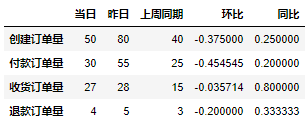``from openpyxl import Workbookfrom openpyxl.utils.dataframe import dataframe_to_rows#创建空工作簿wb = Workbook()ws = wb.active#将DataFrame格式数据转化为openpyxl格式for r in dataframe_to_rows(df_view,index = True,header = True):    ws.append(r)wb.save(r'D:\Data-Science\share\excel-python报表自动化\核心指标_原始.xlsx')``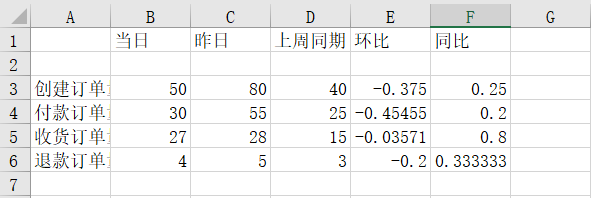``from openpyxl import Workbookfrom openpyxl.utils.dataframe import dataframe_to_rowsfrom openpyxl.styles import colorsfrom openpyxl.styles import Fontfrom openpyxl.styles import PatternFillfrom openpyxl.styles import Border, Sidefrom openpyxl.styles import Alignmentwb = Workbook()ws = wb.activefor r in dataframe_to_rows(df_view,index = True,header = True):    ws.append(r)    #第二行是空的，删除第二行ws.delete_rows(2)#给A1单元格进行赋值ws['A1'] = '指标'#插入一行作为标题行ws.insert_rows(1)ws['A1'] = '电商业务方向 2021/4/11 日报'#将标题行的单元格进行合并ws.merge_cells('A1:F1') #合并单元格#对第1行至第6行的单元格进行格式设置for row in ws[1:6]:    for c in row:        #字体设置        c.font = Font(name = '微软雅黑',size = 12)        #对齐方式设置        c.alignment = Alignment(horizontal = "center")        #边框线设置        c.border = Border(left = Side(border_style = "thin",color = "FF000000"),                   right = Side(border_style = "thin",color = "FF000000"),                   top = Side(border_style = "thin",color = "FF000000"),                   bottom = Side(border_style = "thin",color = "FF000000"))#对标题行和表头行进行特殊设置for row in ws[1:2]:    for c in row:        c.font = Font(name = '微软雅黑',size = 12,bold = True,color = "FFFFFFFF")        c.fill = PatternFill(fill_type = 'solid',start_color='FFFF6100')#将环比和同比设置成百分比格式        for col in ws["E":"F"]:    for r in col:        r.number_format = '0.00%'#调整列宽ws.column_dimensions['A'].width = 13ws.column_dimensions['E'].width = 10#保存调整后的文件        wb.save(r'D:\Data-Science\share\excel-python报表自动化\核心指标.xlsx')``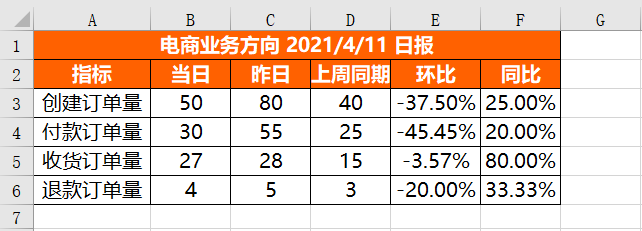## 当日各省份创建订单量情况：

``df_province = pd.DataFrame(df[df['创建日期'] == '2021-04-11'].groupby('省份')['order_id'].count())df_province = df_province.reset_index()df_province = df_province.sort_values(by = 'order_id',ascending = False)df_province = df_province.rename(columns = {'order_id':'创建订单量'})df_province``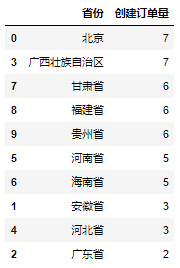``from openpyxl import Workbookfrom openpyxl.utils.dataframe import dataframe_to_rowsfrom openpyxl.styles import colorsfrom openpyxl.styles import Fontfrom openpyxl.styles import PatternFillfrom openpyxl.styles import Border, Sidefrom openpyxl.styles import Alignmentfrom openpyxl.formatting.rule import DataBarRulewb = Workbook()ws = wb.activefor r in dataframe_to_rows(df_province,index = False,header = True):    ws.append(r)#对第1行至第11行的单元格进行设置for row in ws[1:11]:    for c in row:        #字体设置        c.font = Font(name = '微软雅黑',size = 12)        #对齐方式设置        c.alignment = Alignment(horizontal = "center")        #边框线设置        c.border = Border(left = Side(border_style = "thin",color = "FF000000"),                   right = Side(border_style = "thin",color = "FF000000"),                   top = Side(border_style = "thin",color = "FF000000"),                   bottom = Side(border_style = "thin",color = "FF000000"))#设置进度条条件格式rule = DataBarRule(start_type = 'min',end_type = 'max',                    color="FF638EC6", showValue=True, minLength=None, maxLength=None)ws.conditional_formatting.add('B1:B11',rule)#对第1行标题行进行设置for c in ws:    c.font = Font(name = '微软雅黑',size = 12,bold = True,color = "FFFFFFFF")    c.fill = PatternFill(fill_type = 'solid',start_color='FFFF6100')        #调整列宽ws.column_dimensions['A'].width = 17ws.column_dimensions['B'].width = 13#保存调整后的文件     wb.save(r'D:\Data-Science\share\excel-python报表自动化\各省份销量情况.xlsx')``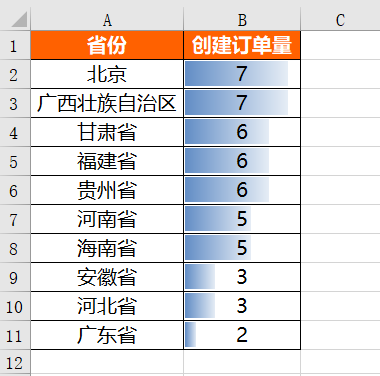## 最近一段时间创建订单量趋势：

``%matplotlib inlineimport matplotlib.pyplot as pltplt.rcParams["font.sans-serif"]='SimHei'#解决中文乱码#设置图表大小plt.figure(figsize = (10,6))df.groupby('创建日期')['order_id'].count().plot()plt.title('4.2 - 4.11 创建订单量分日趋势')plt.xlabel('日期')plt.ylabel('订单量')#将图表保存到本地plt.savefig(r'D:\Data-Science\share\excel-python报表自动化\4.2 - 4.11 创建订单量分日趋势.png')``

``from openpyxl import Workbookfrom openpyxl.drawing.image import Imagewb = Workbook()ws = wb.activeimg = Image(r'D:\Data-Science\share\excel-python报表自动化\4.2 - 4.11 创建订单量分日趋势.png')ws.add_image(img, 'A1')wb.save(r'D:\Data-Science\share\excel-python报表自动化\4.2 - 4.11 创建订单量分日趋势.xlsx')``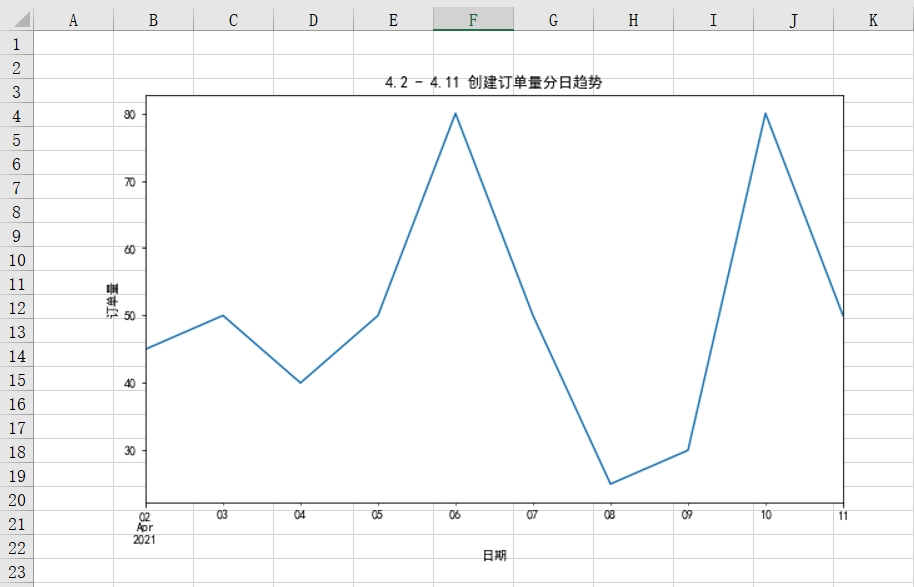# 将不同的结果进行合并

## 将不同的结果合并到同一个Sheet中：

``for r in dataframe_to_rows(df_view,index = True,header = True):    ws.append(r)``

``遍历开始的行 = df_view表占据的行 + 留白的行(一般表与表之间留2行) + 1遍历结束的行 = 遍历开始的行 + df_province表占据的行遍历开始的列 = 1遍历结束的列 = df_province表占据的列``

``for j in range(df_province.shape):    ws.cell(row = df_view.shape + 5,column = 1 + j).value = df_province.columns[r]``

df_province.shape是获取df_province表有多少列，df_view.shape是获取df_view表有多少行。

``#再把具体的值插入for i in range(df_province.shape):    for j in range(df_province.shape):        ws.cell(row = df_view.shape + 6 + i,column = 1 + j).value = df_province.iloc[i,j]``

``#插入图片img = Image(r'D:\Data-Science\share\excel-python报表自动化\4.2 - 4.11 创建订单量分日趋势.png')ws.add_image(img, 'G1')``

``#格式预设#表头字体设置title_Font_style = Font(name = '微软雅黑',size = 12,bold = True,color = "FFFFFFFF")#普通内容字体设置plain_Font_style = Font(name = '微软雅黑',size = 12)Alignment_style = Alignment(horizontal = "center")Border_style = Border(left = Side(border_style = "thin",color = "FF000000"),                   right = Side(border_style = "thin",color = "FF000000"),                   top = Side(border_style = "thin",color = "FF000000"),                   bottom = Side(border_style = "thin",color = "FF000000"))PatternFill_style = PatternFill(fill_type = 'solid',start_color='FFFF6100')``

``#对A1至F6范围内的单元格进行设置for row in ws['A1':'F6']:    for c in row:        c.font = plain_Font_style        c.alignment = Alignment_style        c.border = Border_style#对第1行和第2行的单元格进行设置for row in ws[1:2]:    for c in row:        c.font = title_Font_style        c.fill = PatternFill_style#对E列和F列的单元格进行设置for col in ws["E":"F"]:    for r in col:        r.number_format = '0.00%'#对A9至B19范围内的单元格进行设置for row in ws['A9':'B19']:    for c in row:        c.font = plain_Font_style        c.alignment = Alignment_style        c.border = Border_style#对A9至B9范围内的单元格进行设置for row in ws['A9':'B9']:    for c in row:        c.font = title_Font_style        c.fill = PatternFill_style        #设置进度条rule = DataBarRule(start_type = 'min',end_type = 'max',                    color="FF638EC6", showValue=True, minLength=None, maxLength=None)ws.conditional_formatting.add('B10:B19',rule)#调整列宽ws.column_dimensions['A'].width = 17ws.column_dimensions['B'].width = 13ws.column_dimensions['E'].width = 10``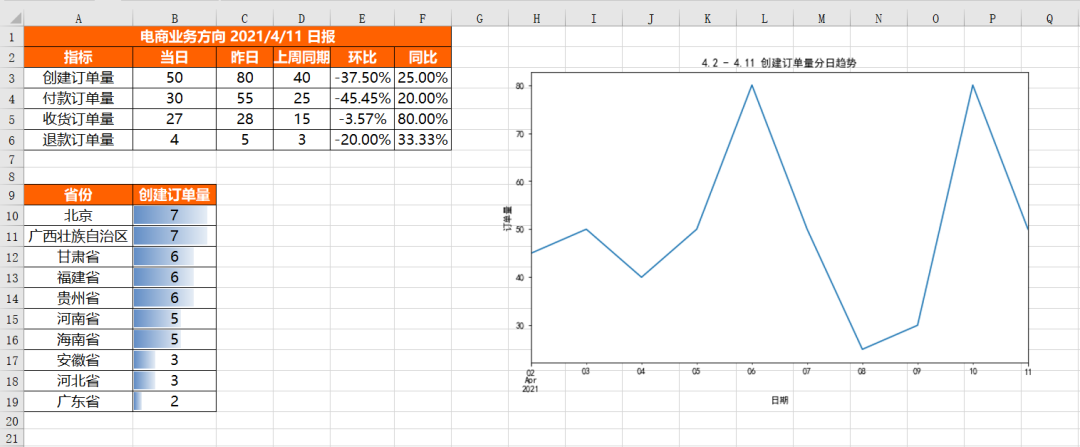## 将不同的结果合并到同一工作簿的不同Sheet中：

``from openpyxl import Workbookfrom openpyxl.utils.dataframe import dataframe_to_rowswb = Workbook()ws = wb.activews1 = wb.create_sheet()ws2 = wb.create_sheet()#更改sheet的名称ws.title = "核心指标" ws1.title = "各省份销情况" ws2.title = "分日趋势" for r1 in dataframe_to_rows(df_view,index = True,header = True):    ws.append(r1)for r2 in dataframe_to_rows(df_province,index = False,header = True):    ws1.append(r2)img = Image(r'D:\Data-Science\share\excel-python报表自动化\4.2 - 4.11 创建订单量分日趋势.png')ws2.add_image(img, 'A1')wb.save(r'D:\Data-Science\share\excel-python报表自动化\多结果合并_多Sheet.xlsx')``